|

# 黑白彰显都市时尚感 东方邦太西德板系列橱柜评测

【导读】现代厨房装饰千变万化，相比老式厨房更强调整体的设计性和功能性，一套好厨房不仅仅烹饪更愉悦，对于烹饪效率也是大有帮助。今天搜房测评专员要为大家介绍东方邦太西德板系列橱柜中一款作品，简约的造型和粉饰有度的图案，书写简单、轻松、快意的现代高品位生活方式，让人在休闲、轻松中享受原汁原味的都市厨房生活情趣，品味烹饪的快乐。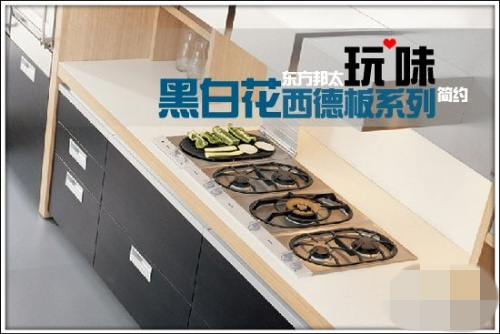如果说红色代表激情，那么蓝色则更显冷静与内敛。深蓝与白色遥相呼应的橱柜面板让人从视觉上就体味到简约，柔美的花卉图案又让它显得不那么冷淡，增添了一分乖巧，凸显主人的性格，彰显都市时尚感。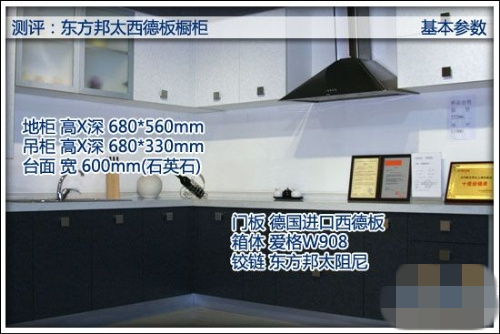测评时间 2011年4月9日 测评地点 上海银都路2988号金盛国际家居33栋3301东方邦太专卖店 测评品牌 东方邦太 测评产品 东方邦太西德板系列橱柜黑白花

产品规格

定制 主要材质 西德板爱格板 产品价格 2560.00元/延米 测评项目 外观 材质 咨询电话 021-61873354/4006308888*2 黑白花玩味简约 东方邦太西德板系列橱柜评测

合金拉手

西德板的拉手采用方块造型，哑光金属效果更符合简约风格。测评员在现场拉开抽屉与柜门非常方便，这是因为东方邦太保留了非常大了接触空间，几乎可以让接触整个手指的长度。同时，银色的拉手在柜面上也增添了层次感。西德板黑白花

西德板相比以前的防火胶合板具有耐高温、阻燃、耐磨、耐冲击、耐腐蚀、耐刮和防潮等一系列特性。在花纹上，则有变化丰富及高科技设计的“面纹”，如柔面、珠面等品种，以及抽象图案、多色彩金属系列等，是一种富有设计感的防火板。从这款东方邦太橱柜上就能看出，柔美的黑白花饰造型让橱柜表面更加时尚动感。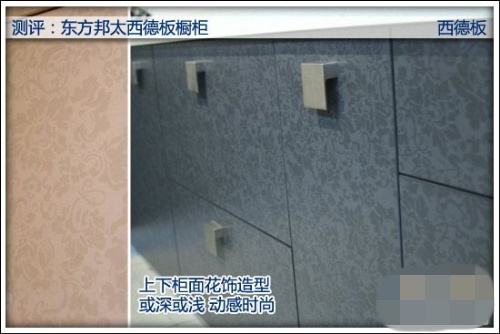爱格板材

这款东方邦太橱柜内部板材使用进口爱格板，爱家板是一种复合型装饰板。在刨花板的基材上覆盖一层经过特殊浸渍处理的、具有抗刻划、耐酸碱的表层，被广泛用于板式家具和厨房家具。爱家板的取材选自生长在欧洲大陆寒带的针叶树种，为使板材有良好的物理性能和再加工性，加工过程中掺和一小部分的锯木屑，克服了天然木材缺点，具有不易变形和稳定的物理性能。生产爱家板的木材来源于末经污染的森林区，整个生产过程中都有严密监控措施，所有的爱家产品都是经过专门国际机构的测定，符合国际环保一级标准E-1的级。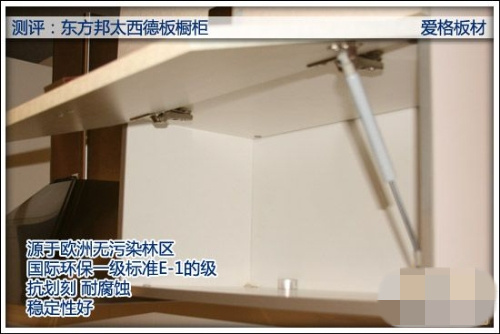合金拉杆增加支撑力

橱柜与水池灶具的结合情况如何也是考量一个橱柜好坏的标准，打开水池下的柜门，测评员发现面板下前后分别有两条横向的合金拉杆，导购告诉我说，横杆的设计在于增加面板对于水池的承重力，这样即使水池中装满水，箱体也不会发生变形，在东方邦太的所有橱柜款式中都广泛的应用到。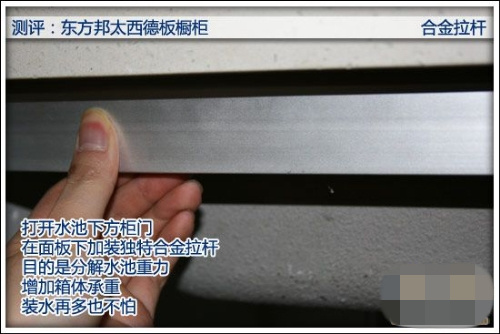防水膜防水防霉变

水池柜体内除了承重措施，当然也少不了防水措施，内部的水管免不了会发生泄露的情况，这时候如果板材直接泡在水里，即使再好的材料也经不起反复折腾，而且也容易霉变。这里东方邦太在底部加了一层镀锡防水膜，这样有些的阻隔漏水渗透，即使不幸的漏水事件真的发生了，也只需要擦干即可，大大延长了使用寿命。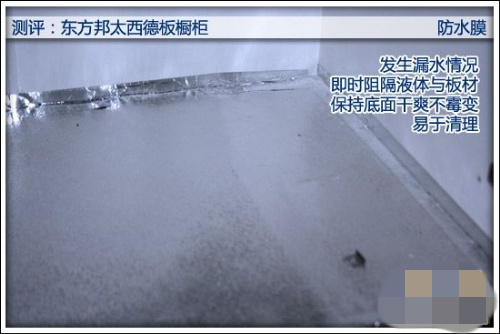黑白花玩味简约 东方邦太西德板系列橱柜评测

多样储藏空间

作为一款橱柜，其基本考量标准自然就是就是储藏能力，这款东方邦太红色西德板橱柜规划了丰富的储物空间，并且搭配不同的材料，比如玻璃移门和磨砂玻璃材质的气压开门，同时也有打开自动滑出托盘的设计来满足不同的需求，美中不足的就是玻璃门没有把手，打开稍有不便。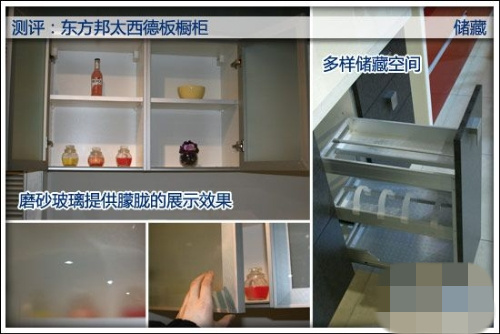石英石台面

石英石的主要成分是二氧化硅，拥有高强度和高硬度等特性。表面相当致密，所以对厨房的酸碱等有极好的抗腐蚀能力，日常使用的液体物质不会渗透其内部，长时间置于表面的液体只需用清水或洁而亮等清洁剂用抹布擦除即可，必要时还可用刀片刮去表面的滞留物。高纯度的石英石由于矿物少更减少了天然石材的辐射危害。测评员在现场用指甲和硬币简单的测试了一下，完全没有留下痕迹。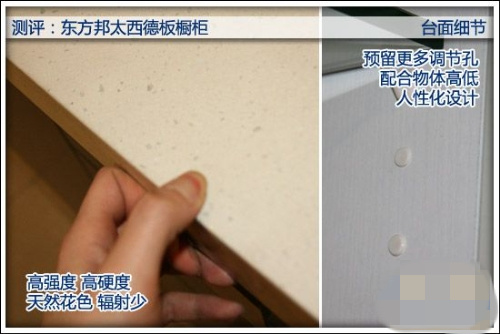五金细节

良好的橱柜五金内件能延长橱柜使用寿命，这款东方邦太橱柜采用自家生产的五金件，测评员在现场打开柜门有一定阻力，并且合上时会自动缓慢关闭。其次在柜内搁板处，测评员注意到还有一个个塑料的圆点，这是特意预留的调节孔，这样可以根据内部存放物体的高低自行调节搁板的高度，非常的人性化。【评测总结】这款东方邦太西德板系列黑白花橱柜整体采用国际品质材料，在质量上当然没的说，包括精细的封边和优秀的加工处理。西德板的运用让它呈现出更加时尚和现代的外观，同时丰富的储藏空间增添了实用性，如果你对于外观和功能都有要求的话，这套橱柜将是不错的选择。

`声明：本文由入驻焦点开放平台的作者撰写，除焦点官方账号外，观点仅代表作者本人，不代表焦点立场错误信息举报电话： 400-099-0099，邮箱：jubao@vip.sohu.com，或点此进行意见反馈，或点此进行举报投诉。`A B C D E F G H J K L M N P Q R S T W X Y Z
A - B - C - D - E
• A
• 鞍山
• 安庆
• 安阳
• 安顺
• 安康
• 澳门
• B
• 北京
• 保定
• 包头
• 巴彦淖尔
• 本溪
• 蚌埠
• 亳州
• 滨州
• 北海
• 百色
• 巴中
• 毕节
• 保山
• 宝鸡
• 白银
• 巴州
• C
• 承德
• 沧州
• 长治
• 赤峰
• 朝阳
• 长春
• 常州
• 滁州
• 池州
• 长沙
• 常德
• 郴州
• 潮州
• 崇左
• 重庆
• 成都
• 楚雄
• 昌都
• 慈溪
• 常熟
• D
• 大同
• 大连
• 丹东
• 大庆
• 东营
• 德州
• 东莞
• 德阳
• 达州
• 大理
• 德宏
• 定西
• 儋州
• 东平
• E
• 鄂尔多斯
• 鄂州
• 恩施
F - G - H - I - J
• F
• 抚顺
• 阜新
• 阜阳
• 福州
• 抚州
• 佛山
• 防城港
• G
• 赣州
• 广州
• 桂林
• 贵港
• 广元
• 广安
• 贵阳
• 固原
• H
• 邯郸
• 衡水
• 呼和浩特
• 呼伦贝尔
• 葫芦岛
• 哈尔滨
• 黑河
• 淮安
• 杭州
• 湖州
• 合肥
• 淮南
• 淮北
• 黄山
• 菏泽
• 鹤壁
• 黄石
• 黄冈
• 衡阳
• 怀化
• 惠州
• 河源
• 贺州
• 河池
• 海口
• 红河
• 汉中
• 海东
• 怀来
• I
• J
• 晋中
• 锦州
• 吉林
• 鸡西
• 佳木斯
• 嘉兴
• 金华
• 景德镇
• 九江
• 吉安
• 济南
• 济宁
• 焦作
• 荆门
• 荆州
• 江门
• 揭阳
• 金昌
• 酒泉
• 嘉峪关
K - L - M - N - P
• K
• 开封
• 昆明
• 昆山
• L
• 廊坊
• 临汾
• 辽阳
• 连云港
• 丽水
• 六安
• 龙岩
• 莱芜
• 临沂
• 聊城
• 洛阳
• 漯河
• 娄底
• 柳州
• 来宾
• 泸州
• 乐山
• 六盘水
• 丽江
• 临沧
• 拉萨
• 林芝
• 兰州
• 陇南
• M
• 牡丹江
• 马鞍山
• 茂名
• 梅州
• 绵阳
• 眉山
• N
• 南京
• 南通
• 宁波
• 南平
• 宁德
• 南昌
• 南阳
• 南宁
• 内江
• 南充
• P
• 盘锦
• 莆田
• 平顶山
• 濮阳
• 攀枝花
• 普洱
• 平凉
Q - R - S - T - W
• Q
• 秦皇岛
• 齐齐哈尔
• 衢州
• 泉州
• 青岛
• 清远
• 钦州
• 黔南
• 曲靖
• 庆阳
• R
• 日照
• 日喀则
• S
• 石家庄
• 沈阳
• 双鸭山
• 绥化
• 上海
• 苏州
• 宿迁
• 绍兴
• 宿州
• 三明
• 上饶
• 三门峡
• 商丘
• 十堰
• 随州
• 邵阳
• 韶关
• 深圳
• 汕头
• 汕尾
• 三亚
• 三沙
• 遂宁
• 山南
• 商洛
• 石嘴山
• T
• 天津
• 唐山
• 太原
• 通辽
• 铁岭
• 泰州
• 台州
• 铜陵
• 泰安
• 铜仁
• 铜川
• 天水
• 天门
• W
• 乌海
• 乌兰察布
• 无锡
• 温州
• 芜湖
• 潍坊
• 威海
• 武汉
• 梧州
• 渭南
• 武威
• 吴忠
• 乌鲁木齐
X - Y - Z
• X
• 邢台
• 徐州
• 宣城
• 厦门
• 新乡
• 许昌
• 信阳
• 襄阳
• 孝感
• 咸宁
• 湘潭
• 湘西
• 西双版纳
• 西安
• 咸阳
• 西宁
• 仙桃
• 西昌
• Y
• 运城
• 营口
• 盐城
• 扬州
• 鹰潭
• 宜春
• 烟台
• 宜昌
• 岳阳
• 益阳
• 永州
• 阳江
• 云浮
• 玉林
• 宜宾
• 雅安
• 玉溪
• 延安
• 榆林
• 银川
• Z
• 张家口
• 镇江
• 舟山
• 漳州
• 淄博
• 枣庄
• 郑州
• 周口
• 驻马店
• 株洲
• 张家界
• 珠海
• 湛江
• 肇庆
• 中山
• 自贡
• 资阳
• 遵义
• 昭通
• 张掖
• 中卫

1室1厅1厨1卫1阳台

1
2
3
4
5

0
1
2

1

1

0
1
2
3报名成功，资料已提交审核A B C D E F G H J K L M N P Q R S T W X Y Z
A - B - C - D - E
• A
• 鞍山
• 安庆
• 安阳
• 安顺
• 安康
• 澳门
• B
• 北京
• 保定
• 包头
• 巴彦淖尔
• 本溪
• 蚌埠
• 亳州
• 滨州
• 北海
• 百色
• 巴中
• 毕节
• 保山
• 宝鸡
• 白银
• 巴州
• C
• 承德
• 沧州
• 长治
• 赤峰
• 朝阳
• 长春
• 常州
• 滁州
• 池州
• 长沙
• 常德
• 郴州
• 潮州
• 崇左
• 重庆
• 成都
• 楚雄
• 昌都
• 慈溪
• 常熟
• D
• 大同
• 大连
• 丹东
• 大庆
• 东营
• 德州
• 东莞
• 德阳
• 达州
• 大理
• 德宏
• 定西
• 儋州
• 东平
• E
• 鄂尔多斯
• 鄂州
• 恩施
F - G - H - I - J
• F
• 抚顺
• 阜新
• 阜阳
• 福州
• 抚州
• 佛山
• 防城港
• G
• 赣州
• 广州
• 桂林
• 贵港
• 广元
• 广安
• 贵阳
• 固原
• H
• 邯郸
• 衡水
• 呼和浩特
• 呼伦贝尔
• 葫芦岛
• 哈尔滨
• 黑河
• 淮安
• 杭州
• 湖州
• 合肥
• 淮南
• 淮北
• 黄山
• 菏泽
• 鹤壁
• 黄石
• 黄冈
• 衡阳
• 怀化
• 惠州
• 河源
• 贺州
• 河池
• 海口
• 红河
• 汉中
• 海东
• 怀来
• I
• J
• 晋中
• 锦州
• 吉林
• 鸡西
• 佳木斯
• 嘉兴
• 金华
• 景德镇
• 九江
• 吉安
• 济南
• 济宁
• 焦作
• 荆门
• 荆州
• 江门
• 揭阳
• 金昌
• 酒泉
• 嘉峪关
K - L - M - N - P
• K
• 开封
• 昆明
• 昆山
• L
• 廊坊
• 临汾
• 辽阳
• 连云港
• 丽水
• 六安
• 龙岩
• 莱芜
• 临沂
• 聊城
• 洛阳
• 漯河
• 娄底
• 柳州
• 来宾
• 泸州
• 乐山
• 六盘水
• 丽江
• 临沧
• 拉萨
• 林芝
• 兰州
• 陇南
• M
• 牡丹江
• 马鞍山
• 茂名
• 梅州
• 绵阳
• 眉山
• N
• 南京
• 南通
• 宁波
• 南平
• 宁德
• 南昌
• 南阳
• 南宁
• 内江
• 南充
• P
• 盘锦
• 莆田
• 平顶山
• 濮阳
• 攀枝花
• 普洱
• 平凉
Q - R - S - T - W
• Q
• 秦皇岛
• 齐齐哈尔
• 衢州
• 泉州
• 青岛
• 清远
• 钦州
• 黔南
• 曲靖
• 庆阳
• R
• 日照
• 日喀则
• S
• 石家庄
• 沈阳
• 双鸭山
• 绥化
• 上海
• 苏州
• 宿迁
• 绍兴
• 宿州
• 三明
• 上饶
• 三门峡
• 商丘
• 十堰
• 随州
• 邵阳
• 韶关
• 深圳
• 汕头
• 汕尾
• 三亚
• 三沙
• 遂宁
• 山南
• 商洛
• 石嘴山
• T
• 天津
• 唐山
• 太原
• 通辽
• 铁岭
• 泰州
• 台州
• 铜陵
• 泰安
• 铜仁
• 铜川
• 天水
• 天门
• W
• 乌海
• 乌兰察布
• 无锡
• 温州
• 芜湖
• 潍坊
• 威海
• 武汉
• 梧州
• 渭南
• 武威
• 吴忠
• 乌鲁木齐
X - Y - Z
• X
• 邢台
• 徐州
• 宣城
• 厦门
• 新乡
• 许昌
• 信阳
• 襄阳
• 孝感
• 咸宁
• 湘潭
• 湘西
• 西双版纳
• 西安
• 咸阳
• 西宁
• 仙桃
• 西昌
• Y
• 运城
• 营口
• 盐城
• 扬州
• 鹰潭
• 宜春
• 烟台
• 宜昌
• 岳阳
• 益阳
• 永州
• 阳江
• 云浮
• 玉林
• 宜宾
• 雅安
• 玉溪
• 延安
• 榆林
• 银川
• Z
• 张家口
• 镇江
• 舟山
• 漳州
• 淄博
• 枣庄
• 郑州
• 周口
• 驻马店
• 株洲
• 张家界
• 珠海
• 湛江
• 肇庆
• 中山
• 自贡
• 资阳
• 遵义
• 昭通
• 张掖
• 中卫• 手机• 分享
• 设计
免费设计
• 计算器
装修计算器
• 入驻
合作入驻
• 联系
联系我们
• 置顶
返回顶部